# 玩转YY老师服务器(下)之自动化刷题脚本编写

### 玩转YY老师服务器(下)之自动化刷题脚本编写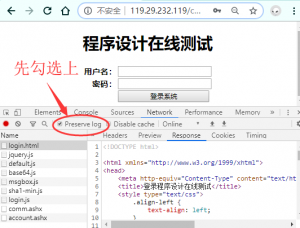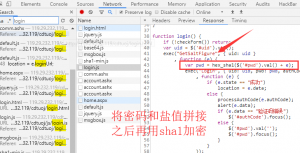```import requests
from hashlib import sha1

s = requests.session()
url = 'http://119.29.232.119/cdtuoj/account.ashx'
data = {'uid': uid,'method': 'GetSaltFigure'}
r = s.post(url, data=data)
pwd = sha1(pwd.encode('utf-8')).hexdigest()
data = {'uid': uid,'pwd': pwd,'method': 'Login'}
r = s.post(url, data=data)
print('yy老师服务器登录成功！')
return s
else:
print('用户名或密码错误，请检查后重试！')
sys.exit(1)``````def get_info(s):
for i in range(1,6):
data = {'pager_newPageIndex': str(i)}
r = s.post('http://119.29.232.119/cdtuoj/home.aspx', data=data, verify=False)
soup = BeautifulSoup(r.text, 'lxml')
table = soup.find_all('table')
tr = table.find_all('tr')[1:]
for i in tr:
td = i.find_all('td')
num = td.string
title = td.string
qid = i.a['href'].split('=')[-1]
yield (num,title,qid)```

```def save_exe(num,title,qid,s):
os.chdir('E:/yy/')
filedir = '第'+num +'题'+ title.replace(' ','')
os.mkdir(filedir)
os.chdir(filedir)
filename = 'question{}.exe'.format(qid)
with open(filename,'wb')as f:
f.write(r.content)
print(filedir+'的例程下载完成！')
return filename```• `-A` 让ida自动运行，不需要人工干预。也就是在处理的过程中不会弹出交互窗口，但是如果从来没有使用过ida那么许可协议的窗口无论你是否使用这个参数都将会显示。
• `-c` 参数会删除所有与参数中指定的文件相关的数据库，并且生成一个新的数据库。
• `-S` 参数用于指定ida在分析完数据之后执行的idc脚本，该选项和参数之间没有空格，并且搜索目录为ida目录下的idc文件夹。
• `-B` 参数指定批量模式，等效于-A –c –Sscript.py.在分析完成后会自动生成相关的数据库和asm代码。并且在最后关闭ida，以保存新的数据库。
• `-h` 显示ida的帮助文档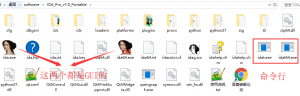#### aspx网站中form使用到了__VIEWSTATE、__EVENTVALIDATION来验证的提交

__VIEWSTATE
ViewState是ASP.NET中用来保存WEB控件回传时状态值一种机制。在WEB窗体(FORM)的设置为runat=”server”,这个窗体(FORM)会被附加一个隐藏的属性_VIEWSTATE。_VIEWSTATE中存放了所有控件在ViewState中的状态值。
__EVENTVALIDATION只是用来验证事件是否从合法的页面发送，只是一个数字签名，所以一般很短。
“id”属性为“__EVENTVALIDATION”的隐藏字段是ASP.NET 2.0的新增的安全措施。该功能可以阻止由潜在的恶意用户从浏览器端发送的未经授权的请求.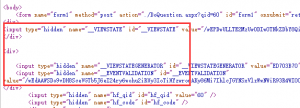```def get_hideinfo(url,s):
d = {}
r = s.get(url)
soup = BeautifulSoup(r.text, 'lxml')
tag = soup.find_all('input')
for i in tag:
if '__' in i['name']:
d[i['name']] = i['value']
return d```

```def post_code(s,qid):
url = 'http://119.29.232.119/cdtuoj/DoQuestion.aspx?qid={}'.format(qid)
d = get_hideinfo(url,s)
with open('question{}.cpp'.format(qid),'r')as f:
code_base64 = str(base64.b64encode(code.encode('utf-8')),'utf-8')
data = {'hf_qid': qid,'hf_code': code_base64}
data.update(d)
r = s.post(url ,data=data,allow_redirects=False)
print('正在提交代码等待中......')
time.sleep(5)
data = {'qid':qid,'method': 'GetStatusForUser'}
r = s.post('http://119.29.232.119/cdtuoj/QuestionSvr.ashx' ,data=data)
print('当前提交代码状态为： '+state)```

```def get_exception(func):
def new(*args,**kwargs):
try:
return func(*args,**kwargs)
except Exception as e:
print('代码报错已忽略请自行debug，错误信息为:\n',repr(e),traceback.print_exc())
return new```

```import os
import sys
import json
import time
import base64
import requests
import traceback
from hashlib import sha1
from bs4 import BeautifulSoup

def get_exception(func):
def new(*args,**kwargs):
try:
return func(*args,**kwargs)
except Exception as e:
print('代码报错已忽略请自行debug，错误信息为:\n',repr(e),traceback.print_exc())
return new

def user_input():
uid = input('请输入你的学号信息：').strip()
pwd = input('请输入你的密码：').strip()
return uid,pwd

def check_dir():
path = 'E:/yy/'
if not os.path.exists(path):os.mkdir(path)
os.chdir(path)

@get_exception
s = requests.session()
url = 'http://119.29.232.119/cdtuoj/account.ashx'
data = {'uid': uid,'method': 'GetSaltFigure'}
r = s.post(url, data=data)
pwd = sha1(pwd.encode('utf-8')).hexdigest()
data = {'uid': uid,'pwd': pwd,'method': 'Login'}
r = s.post(url, data=data)
print('yy老师服务器登录成功！')
return s
else:
print('用户名或密码错误，请检查后重试！')
sys.exit(1)

@get_exception
def get_info(s):
for i in range(1,6):
data = {'pager_newPageIndex': str(i)}
r = s.post('http://119.29.232.119/cdtuoj/home.aspx', data=data, verify=False)
soup = BeautifulSoup(r.text, 'lxml')
table = soup.find_all('table')
tr = table.find_all('tr')[1:]
for i in tr:
td = i.find_all('td')
num = td.string
title = td.string
qid = i.a['href'].split('=')[-1]
yield (num,title,qid)

@get_exception
def save_exe(num,title,qid,s):
os.chdir('E:/yy/')
filedir = '第'+num +'题'+ title.replace(' ','')
os.mkdir(filedir)
os.chdir(filedir)
filename = 'question{}.exe'.format(qid)
with open(filename,'wb')as f:
f.write(r.content)
print(filedir+'的例程下载完成！')
return filename

@get_exception
def decompile(filename):
args = '-c -A -Syy.py '
cmd = IDA_path + args + filename
os.system(cmd)
print(os.path.basename(filename)+' 已反编译生成为cpp文件！')

def get_hideinfo(url,s):
d = {}
r = s.get(url)
soup = BeautifulSoup(r.text, 'lxml')
tag = soup.find_all('input')
for i in tag:
if '__' in i['name']:
d[i['name']] = i['value']
return d

@get_exception
def post_code(s,qid):
url = 'http://119.29.232.119/cdtuoj/DoQuestion.aspx?qid={}'.format(qid)
d = get_hideinfo(url,s)
with open('question{}.cpp'.format(qid),'r')as f:
code_base64 = str(base64.b64encode(code.encode('utf-8')),'utf-8')
data = {'hf_qid': qid,'hf_code': code_base64}
data.update(d)
r = s.post(url ,data=data,allow_redirects=False)
print('正在提交代码等待中......')
time.sleep(5)
data = {'qid':qid,'method': 'GetStatusForUser'}
r = s.post('http://119.29.232.119/cdtuoj/QuestionSvr.ashx' ,data=data)
print('当前提交代码状态为： '+state)

if __name__ == '__main__':
check_dir()
info = user_input()
for i in get_info(s):
filename = save_exe(*i,s)
decompile(filename)
post_code(s,i)```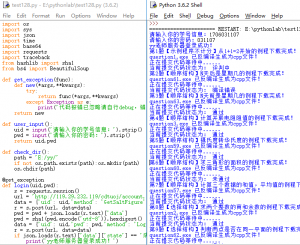微信赞赏支付宝赞赏### 4条评论###### islot.cn 发布于11:25 下午 - 5月 15, 2020###### jungle 发布于12:35 上午 - 10月 18, 2019###### CxMiao 发布于5:26 下午 - 10月 9, 2019